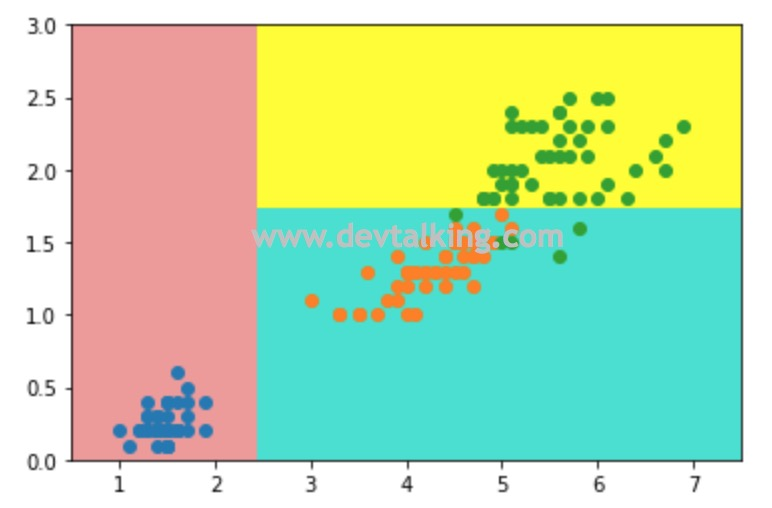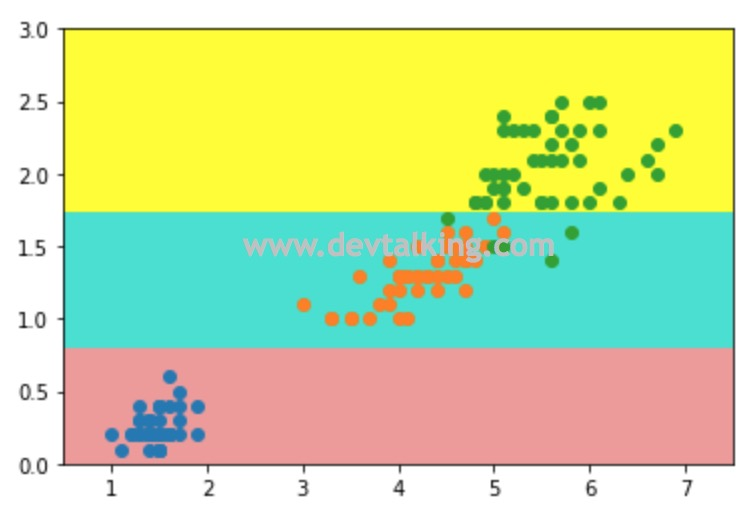基尼系数

$$G = 1 - \sum_{i=1}^k p_i^2$$

$$\{\frac 1 3,\frac 1 3,\frac 1 3\}$$

$$G = 1-(\frac 1 3)^2-(\frac 1 3)^2-(\frac 1 3)^2=0.6666$$

$$\{\frac 1 {10},\frac 2 {10},\frac 7 {10}\}$$

$$G = 1-(\frac 1 {10})^2-(\frac 2 {10})^2-(\frac 7 {10})^2=0.46$$

信息熵和基尼系数的比较

• 信息熵的计算比基尼系数的稍慢一些，因为信息熵的公式里是要求$log$的，而基尼系数公式中只是平方求和而已。
• Scikit Learn中的决策树默认使用基尼系数方式，所以当我们不传入criterion参数时，默认使用gini方式。
• 信息熵和基尼系数没有特别的效果优劣。只是大家需要了解决策树根节点划分的方式原理。

决策树中的超参数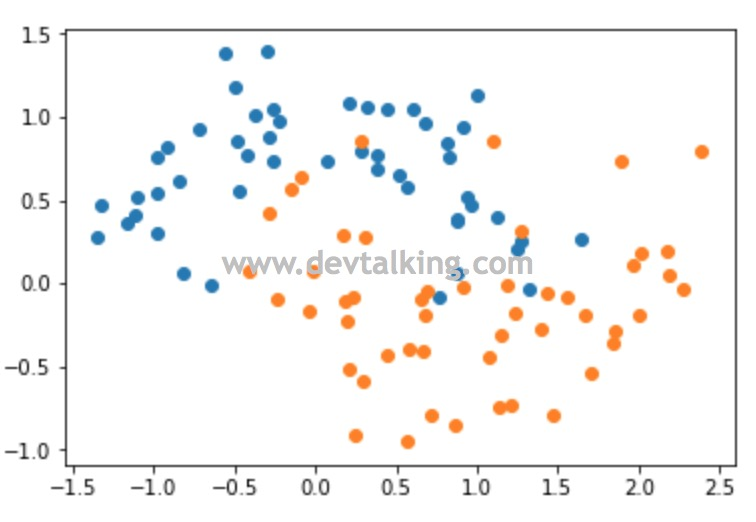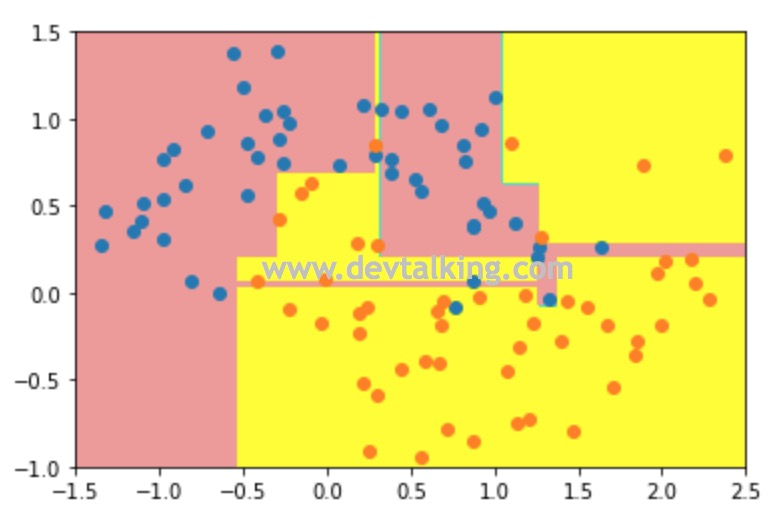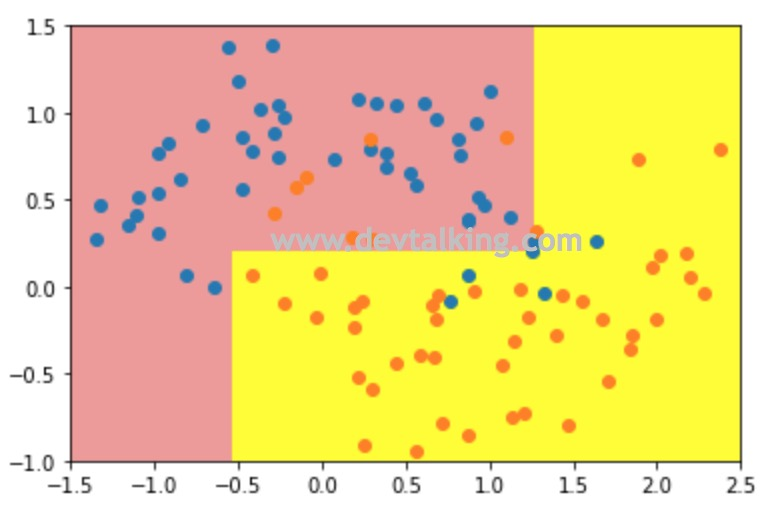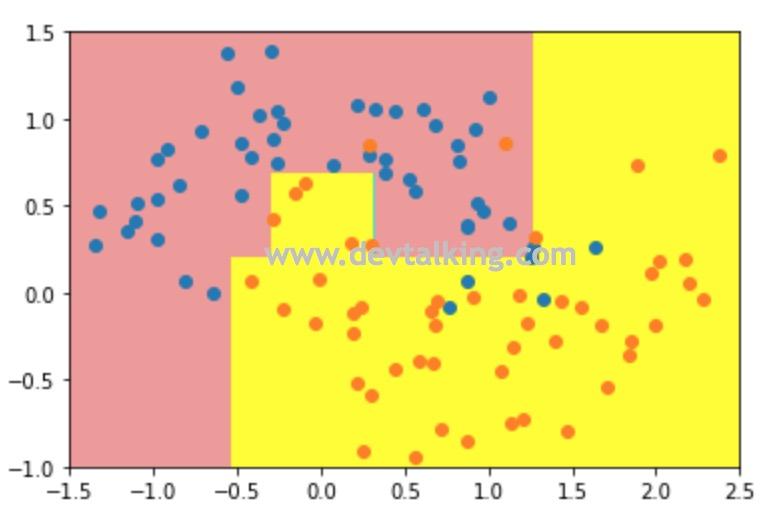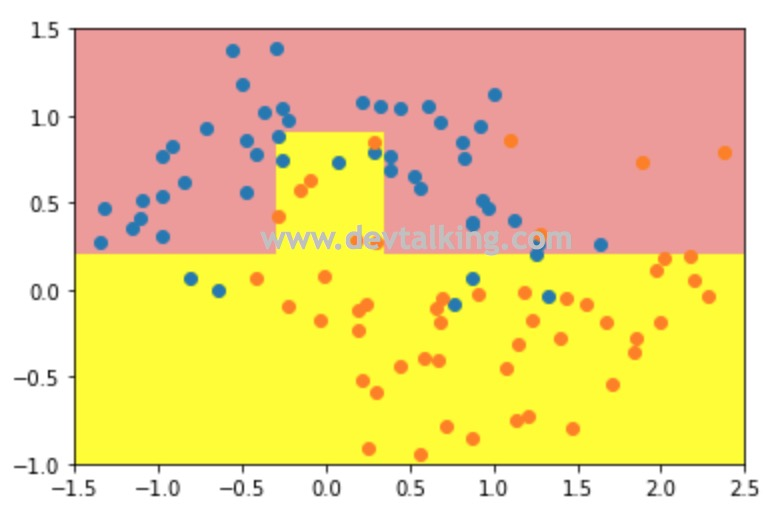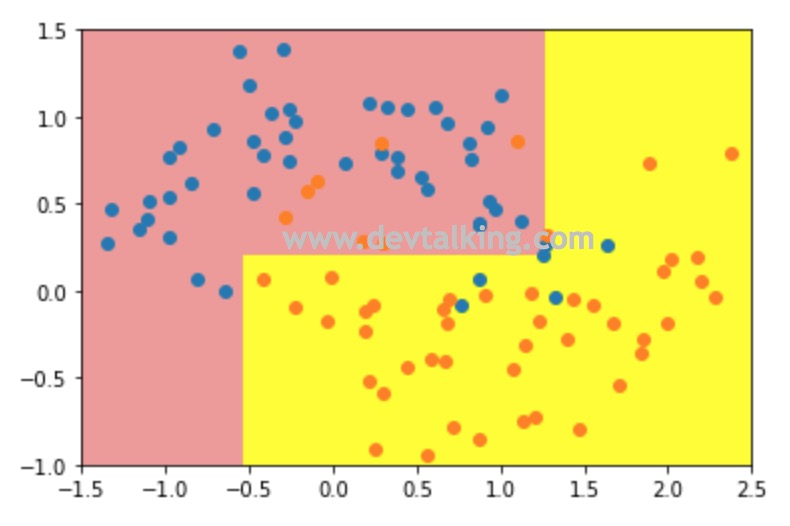CART

CART是Classification And Regression Tree的缩写，字面意思已经很明确了，分类和回归树，说明我们这篇笔记中介绍的基于信息熵和基尼系数划分方式的决策树既可以解决分类问题，还可以解决回归问题。Scikit Learn中封装的决策树也是CART，当然还有一些其他实现方式的决策树，大家有兴趣可以在网上查阅ID3，C4.5，C5.0等实现方式的决策树。

决策树的缺陷

决策边界的局限性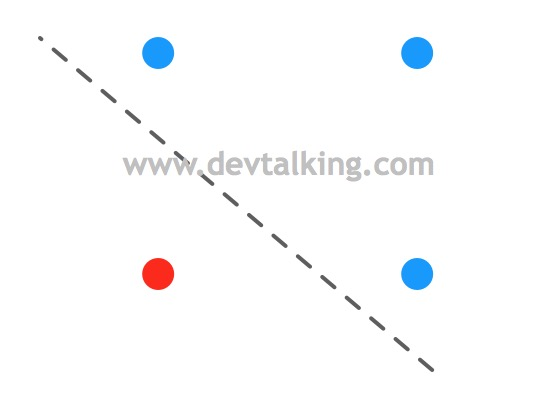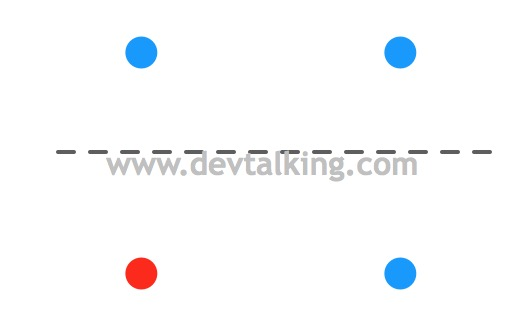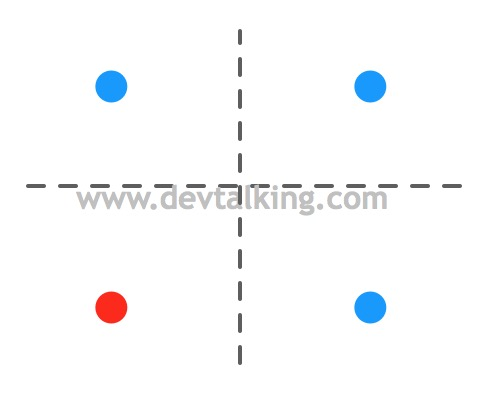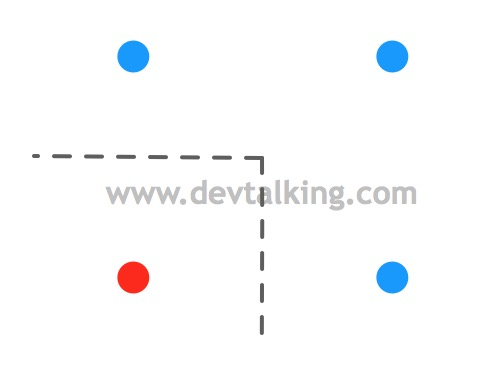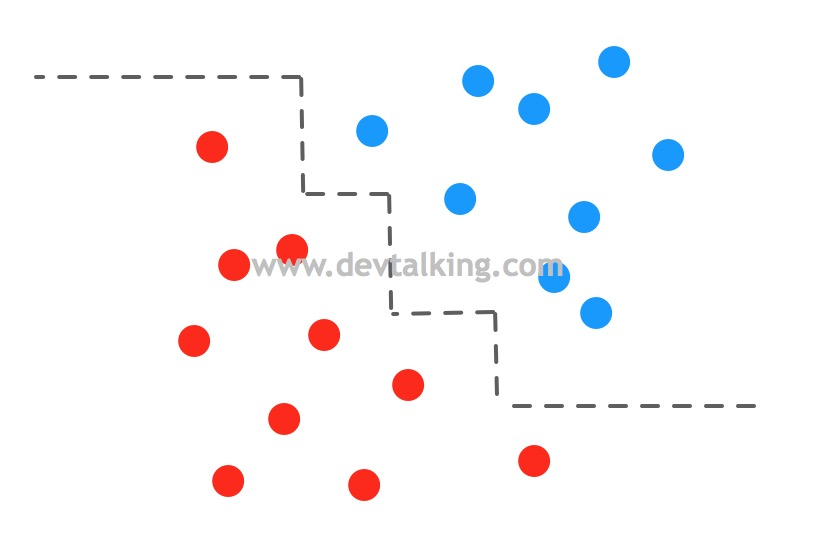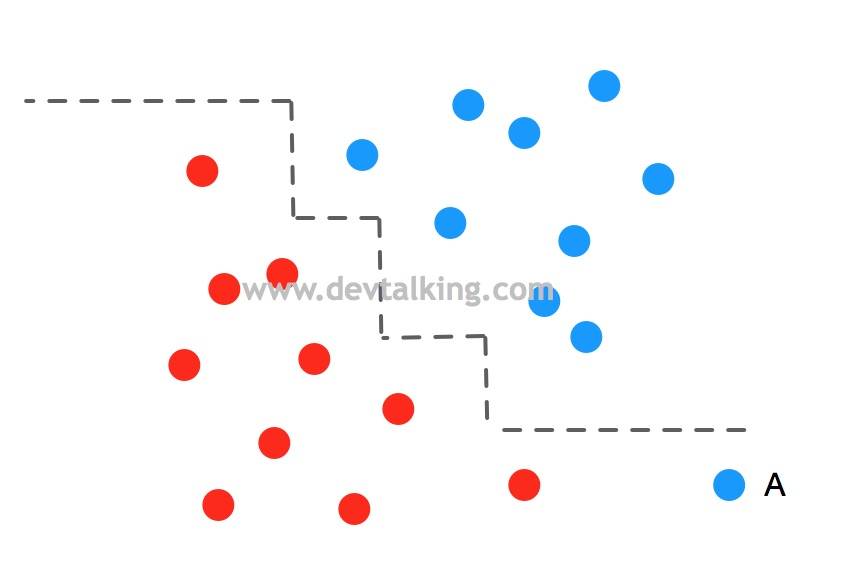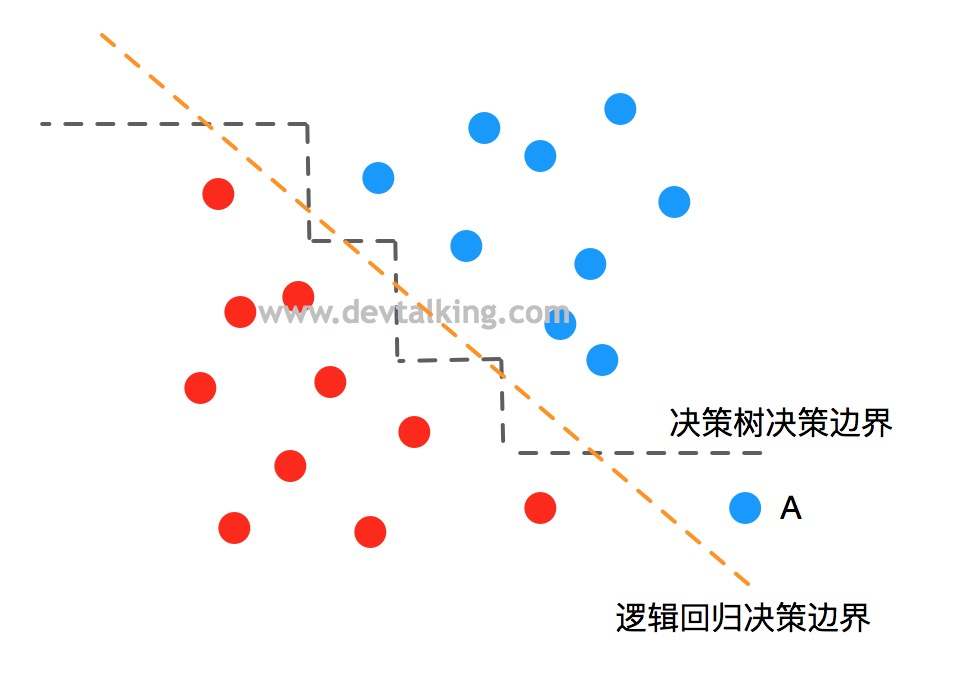对数据敏感